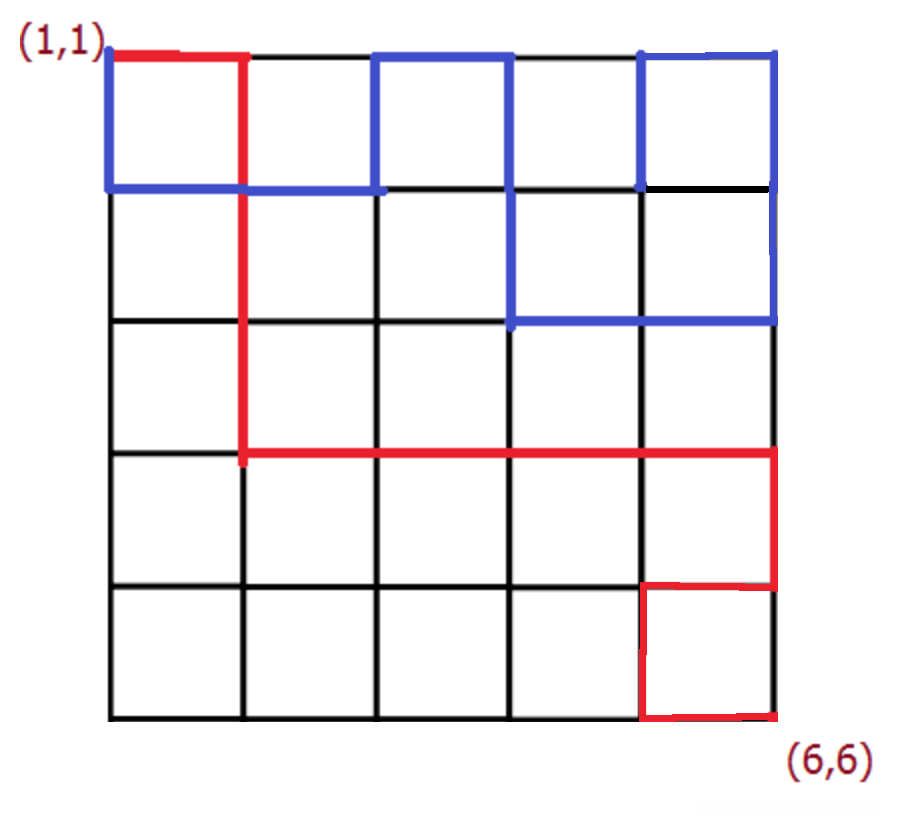Computer Science

# Monte-Carlo

Brilli the ant is trying to get from point $$A$$ to point $$B$$ in a grid. The coordinates of point $$A$$ is $$(1,1)$$ (this is top left corner), and the coordinates of point $$B$$ is $$(n,n)$$ (this is bottom right corner, $$n$$ is the size of the grid).

Once Brilli starts moving, there are four options, it can go left, right, up or down (no diagonal movement allowed). If any of these four options satisfy the following:

• The new point should still be within the boundaries of the $$n \times n$$ grid
• The new point should not be visited previously.

If $$P$$ is the probability of Brilli the ant reaching point $$B$$ for a $$6 \times 6$$ grid, use Monte Carlo simulation to compute $$P$$. Pick the answer closest to $$P$$ in value.Details and Assumptions

• Assume $$10,000$$ simulations are sufficient enough to compute $$P$$.

Write a function that uses a Monte Carlo simulation to find the probability of a run of $$4$$ consecutive tails out of ten flips of a fair coin, and then returns that probability.

What is the first number after the decimal point of the output value?

Details and Assumptions

Assume that $$10,000$$ trials are sufficient to provide an accurate answer.

Three points are chosen uniformly at random from the perimeter of a unit circle. Use Monte Carlo simulation to compute the probability of the points forming an acute triangle, what is the probability?

Details and Assumptions

• Assume $$10,000$$ simulations are sufficient.
×

Problem Loading...

Note Loading...

Set Loading...